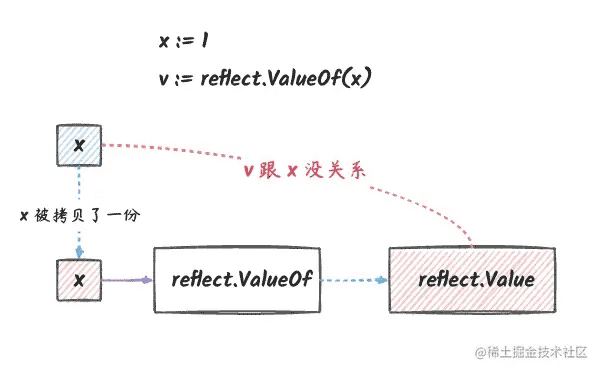﻿ go reflect要不要传指针原理详解_Golang_脚本之家
Golang# go reflect要不要传指针原理详解

## 正文

```var a = 1
v1 := reflect.ValueOf(a)
v2 := reflect.ValueOf(&a)
fmt.Println(v1.Int())        // 1
fmt.Println(v2.Elem().Int()) // 1
```

## 什么时候传递指针？

• 是否要修改变量的值，要修改就要用指针
• 结构体类型：是否要修改结构体里的字段，要修改就要用指针
• 结构体类型：是否要调用指针接收值方法，要调用就要用指针
• 对于 `chan``map``slice` 类型，我们传递值和传递指针都可以修改其内容
• 对于非 `interface` 类型，传递给 `TypeOf``ValueOf` 的时候都会转换为 `interface` 类型，如果本身就是 `interface` 类型，则不需转换。
• 指针类型不可修改，但是可以修改指针指向的值。（`v := reflect.ValueOf(&a)``v.CanSet()``false``v.Elem().CanSet()``true`
• 字符串：我们可以对字符串进行替换，但不能修改字符串的某一个字符

## 1. 通过传递指针修改变量的值

### 传值无法修改变量本身

```x := 1
v := reflect.ValueOf(x)
```### 传指针可以修改变量

```x := 1
v := reflect.ValueOf(&amp;x).Elem()
```## 2. 通过传递指针修改结构体的字段

```type person struct {
Name string
Age  int
}
p := person{
Name: "foo",
Age:  30,
}
// v 本质上是指向 p 的指针
v := reflect.ValueOf(&p)
// v.CanSet() 为 false，v 是指针，指针本身是不能修改的
// v.Elem() 是 p 本身，是可以修改的
fmt.Println(v.Elem().FieldByName("Name").CanSet()) // true
fmt.Println(v.Elem().FieldByName("Age").CanSet())  // true
```## 3. 结构体：获取指针接收值方法

```type person struct {
}
func (p person) M1() {
}
func (p *person) M2() {
}
func TestPerson(t *testing.T) {
p := person{}
v1 := reflect.ValueOf(p)
v2 := reflect.ValueOf(&p)
assert.Equal(t, 1, v1.NumMethod())
assert.Equal(t, 2, v2.NumMethod())
// v1 和 v2 都有 M1 方法
assert.True(t, v1.MethodByName("M1").IsValid())
assert.True(t, v2.MethodByName("M1").IsValid())
// v1 没有 M2 方法
// v2 有 M2 方法
assert.False(t, v1.MethodByName("M2").IsValid())
assert.True(t, v2.MethodByName("M2").IsValid())
}
```## 4. 变量本身包含指向数据的指针

### 通过值反射对象修改 chan、map 和 slice```func TestPointer1(t *testing.T) {
// 数组需要传递引用才能修改其元素
arr := int{1, 2, 3}
v1 := reflect.ValueOf(&arr)
v1.Elem().Index(1).SetInt(100)
assert.Equal(t, 100, arr)
// chan 传值也可以修改其元素
ch := make(chan int, 1)
v2 := reflect.ValueOf(ch)
v2.Send(reflect.ValueOf(10))
assert.Equal(t, 10, <-ch)
// map 传值也可以修改其元素
m := make(map[int]int)
v3 := reflect.ValueOf(m)
v3.SetMapIndex(reflect.ValueOf(1), reflect.ValueOf(10))
assert.Equal(t, 10, m)
// slice 传值也可以修改其元素
s := []int{1, 2, 3}
v4 := reflect.ValueOf(s)
v4.Index(1).SetInt(20)
assert.Equal(t, 20, s)
}
```

### slice 反射对象扩容的影响```func TestPointer1(t *testing.T) {
s := []int{1, 2, 3}
v4 := reflect.ValueOf(s)
v4.Index(1).SetInt(20)
assert.Equal(t, 20, s)
// 这里发生了扩容
// v5 的 array 跟 s 的 array 指向的是不同的内存区域了。
v5 := reflect.Append(v4, reflect.ValueOf(4))
fmt.Println(s) // [1 20 3]
fmt.Println(v5.Interface().([]int)) // [1 20 3 4]
// 这里修改 v5 的时候影响不到 s 了
v5.Index(1).SetInt(30)
fmt.Println(s) // [1 20 3]
fmt.Println(v5.Interface().([]int)) // [1 30 3 4]
}
```

### slice 容量够的话是不是就可以正常追加元素了？

```func TestPointer000(t *testing.T) {
s1 := make([]int, 3, 6)
s1 = 1
s1 = 2
s1 = 3
fmt.Println(s1) // [1 2 3]
v6 := reflect.ValueOf(s1)
v7 := reflect.Append(v6, reflect.ValueOf(4))
// 虽然 s1 的容量足够大，但是 s1 还是看不到追加的元素
fmt.Println(s1)                     // [1 2 3]
fmt.Println(v7.Interface().([]int)) // [1 2 3 4]
// s1 和 s2 底层数组还是同一个
// array1 是 s1 底层数组的内存地址
s2 := v7.Interface().([]int)
// array2 是 s2 底层数组的内存地址
assert.Equal(t, array1, array2)
// 这是因为 s1 的长度并没有发生改变，
// 所以 s1 看不到追加的那个元素
fmt.Println(len(s1), cap(s1)) // 3 6
fmt.Println(len(s2), cap(s2)) // 4 6
}
```

### map 也不能通过值反射对象来修改其元素。

`slice` 类似，通过 `map` 的值反射对象来追加元素的时候，同样可能导致扩容， 扩容之后，保存数据的内存区域会发生改变。

### chan 没有追加

`chan``slice``map` 有个不一样的地方，它的长度是我们创建 `chan` 的时候就已经固定的了， 因此，不存在扩容导致指向内存区域发生改变的问题。

### 结构体字段包含指针的情况

```type person struct {
Name *string
}
func TestPointerPerson(t *testing.T) {
name := "foo"
p := person{Name: &name}
v := reflect.ValueOf(p)
fmt.Println(v.Field(0).Elem().CanSet())
name1 := "bar"
v.Field(0).Elem().Set(reflect.ValueOf(name1))
// p 的 Name 字段已经被成功修改
fmt.Println(*p.Name)
}
```

## 5. interface 类型处理

```// v1 跟 v2 都拿到了 a 的拷贝
var a = 1
v1 := reflect.ValueOf(a)
var b interface{} = a
v2 := reflect.ValueOf(b)
```

```assert.Equal(t, v1.Kind(), v2.Kind())
assert.Equal(t, v1.CanSet(), v2.CanSet())
assert.Equal(t, v1.Interface(), v2.Interface())
```

```// v1 跟 v2 都拿到了 a 的指针
var a = 1
v1 := reflect.ValueOf(&a)
var b interface{} = &a
v2 := reflect.ValueOf(b)
```

```assert.Equal(t, v1.Kind(), v2.Kind())
assert.Equal(t, v1.Elem().Kind(), v2.Elem().Kind())
assert.Equal(t, v1.Interface(), v2.Interface())
assert.Equal(t, v1.Elem().Interface(), v2.Elem().Interface())
```

### interface 底层类型是值

`interface` 类型的底层类型是值的时候，我们将其传给 `reflect.ValueOf` 跟直接传值是一样的。 是没有办法修改 `interface` 底层数据的值的（除了指针类型字段，因为反射对象也拿到了指针字段的地址）：

```type person struct {
Name *string
}
func TestInterface1(t *testing.T) {
name := "foo"
p := person{Name: &name}
// v 拿到的是 p 的拷贝
// 下面两行等价于 v := reflect.ValueOf(p)
var i interface{} = p
v := reflect.ValueOf(i)
assert.Equal(t, reflect.Struct, v.Kind())
}
```### interface 底层类型是指针

```func TestInterface(t *testing.T) {
var a = 1
v1 := reflect.ValueOf(&a)
var b interface{} = &a
v2 := reflect.ValueOf(b)
// v1 和 v2 本质上都接收了一个 interface 参数，
// 这个 interface 参数的数据部分都是 &a
v1.Elem().SetInt(10)
assert.Equal(t, 10, a)
// 通过 v1 修改 a 的值，v2 也能看到
assert.Equal(t, 10, v2.Elem().Interface())
// 同样的，通过 v2 修改 a 的值，v1 也能看到
v2.Elem().SetInt(20)
assert.Equal(t, 20, a)
assert.Equal(t, 20, v1.Elem().Interface())
}
```

### 不要再对接口类型取地址

```func TestInterface(t *testing.T) {
var a = 1
var i interface{} = a
v1 := reflect.ValueOf(&a)
v2 := reflect.ValueOf(&i)
// v1 和 v2 的类型都是 reflect.Ptr
assert.Equal(t, reflect.Ptr, v1.Kind())
assert.Equal(t, reflect.Ptr, v2.Kind())
// 但是两者的 Elem() 类型不同，
// v1 的 Elem() 是 reflect.Int，
// v2 的 Elem() 是 reflect.Interface
assert.Equal(t, reflect.Int, v1.Elem().Kind())
assert.Equal(t, reflect.Interface, v2.Elem().Kind())
}
```

```assert.Equal(t, "<*interface {} Value>", v2.String())
assert.Equal(t, "<interface {} Value>", v2.Elem().String())
assert.Equal(t, "<int Value>", v2.Elem().Elem().String())
```• `i` 是接口类型，它的数据部分是 `a` 的拷贝，它的类型部分是 `int` 类型。
• `&i` 是指向接口的指针，它指向了上图的 `eface`
• `v2` 是指向 `eface` 的指针的反射对象。
• 最终，我们通过 `v2` 找到 `i` 这个接口，然后通过 `i` 找到 `a` 这个变量的拷贝

## 6. 指针类型反射对象不可修改其指向地址

```func TestPointer(t *testing.T) {
var a = 1
var b = &a
v := reflect.ValueOf(b)
assert.False(t, v.CanSet())
assert.True(t, v.Elem().CanSet())
}
```• `v` 是指向 `&a` 的指针的反射对象。
• 通过这个反射对象的 `Elem()` 方法，我们可以找到原始的变量 `a`
• 反射对象本身不能修改，但是它的 `Elem()` 方法返回的反射对象可以修改。

## 7. 反射也不能修改字符串中的字符

```type StringHeader struct {
Data uintptr
Len  int
}
```
• `Data` 是指向字符串的指针。
• `Len` 是字符串的长度（单位为字节）。

### 相同的字符串只有一个实例

```s1 := "hello"
s2 := "hello"
```

```v1 := (*reflect.StringHeader)(unsafe.Pointer(&s1))
// 两个字符串实例保存字符串的内存地址是一样的
assert.Equal(t, v1.Data, v2.Data)
```### 字符串本身可以替换

```func TestStirng(t *testing.T) {
s := "hello"
v := reflect.ValueOf(&s)
fmt.Println(v.Elem().CanSet())
v.Elem().SetString("world")
fmt.Println(s) // world
}
```

```func TestStirng(t *testing.T) {
s := "hello"
v := reflect.ValueOf(&s)
v.Elem().SetString("world")
// 修改之后，实际保存字符串的内存地址发生了改变
}
```## 总结

• 如果我们需要通过反射对象来修改变量的值，那么我们必须得有办法拿到变量实际存储的内存地址。这种情况下，很多时候都是通过传递指针给 `reflect.ValueOf()` 方法来实现的。
• 但是对于 `chan``map``slice` 或者其他类似的数据结构，它们通过指针来引用实际存储数据的内存，这种数据结构是通过通过传值给 `reflect.ValueOf()` 方法来实现修改其中的元素的。因为这些数据结构的数据部分可以通过指针的拷贝来修改。
• 但是 `map``slice` 有可能会扩容，如果通过反射对象来追加元素，可能导致追加失败。这是因为，通过反射对象追加元素的时候，如果扩容了，那么原来的内存地址就会失效，这样我们其实就修改不了原来的 `map``slice` 了。
• 同样的，结构体传值来创建反射对象的时候，如果其中有指针类型的字段，那么我们也可以通过指针来修改其中的元素。但是其他字段也还是修改不了的。
• 如果我们创建反射对象的参数是 `interface` 类型，那么能不能修改元素的变量还是取决于我们这个 `interface` 类型变量的数据部分是值还是指针。如果 `interface` 变量中存储的是值，那么我们就不能修改其中的元素了。如果 `interface` 变量中存储的是指针，就可以修改。
• 我们无法修改字符串的某一个字符，通过反射也不能，因为字符串本身是不可变的。不同的 `stirng` 类型的变量，如果它们的值是一样的，那么它们会共享实际存储字符串的内存。
• 但是我们可以直接用一个新的字符串替代旧的字符串。